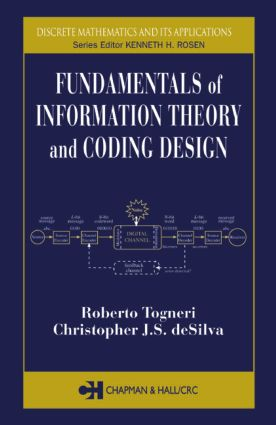# Fundamentals of Information Theory and Coding Design

## 1st Edition

Chapman and Hall/CRC

385 pages | 72 B/W Illus.

##### Purchasing Options:\$ = USD
Hardback: 9781584883104
pub: 2003-01-13
SAVE ~\$28.00
\$140.00
\$112.00
x
eBook (VitalSource) : 9780429225284
pub: 2003-01-13
from \$70.00

FREE Standard Shipping!

### Description

Books on information theory and coding have proliferated over the last few years, but few succeed in covering the fundamentals without losing students in mathematical abstraction. Even fewer build the essential theoretical framework when presenting algorithms and implementation details of modern coding systems.

Without abandoning the theoretical foundations, Fundamentals of Information Theory and Coding Design presents working algorithms and implementations that can be used to design and create real systems. The emphasis is on the underlying concepts governing information theory and the mathematical basis for modern coding systems, but the authors also provide the practical details of important codes like Reed-Solomon, BCH, and Turbo codes. Also setting this text apart are discussions on the cascading of information channels and the additivity of information, the details of arithmetic coding, and the connection between coding of extensions and Markov modelling.

Complete, balanced coverage, an outstanding format, and a wealth of examples and exercises make this an outstanding text for upper-level students in computer science, mathematics, and engineering and a valuable reference for telecommunications engineers and coding theory researchers.

### Reviews

"This book is one of the few (if not the only) texts that comprehensively deal with both the fundamentals of information theory and coding theory. The extensive use of worked examples throughout the text, especially in the more theoretical chapters 6 and 7, will greatly aid students understanding of the principles and methods discussed. The highlighting of definitions, theorems and results allows students to quickly identify and remember the important concepts. The exercise sets at the end of each chapter are quite complete with the routine questions balanced by more challenging and interesting questions. The introduction to the main concepts of abstract algebra used for the design of advanced error detecting and error correcting codes is rigorous, complete and the use of many worked examples makes it one of the best I have seen. The material is also quite extensive with discussions on additivity of mutual information, implementation details of arithmetic coding, rate distortion theory and the important Hamming and Gilbert bounds for channel codes. Overall, this is an excellent and timely textbook for senior undergraduate courses in information and coding theory for students in computer science, mathematics, and engineering."

-Li Deng, Ph.D., Senior Researcher, Microsoft Research, Redmond, WA, USA

ENTROPY AND INFORMATION

Structure

Structure in Randomness

First Concepts of Probability Theory

Surprise and Entropy

Units of Entropy

The Minimum and Maximum Values of Entropy

A Useful Inequality

Joint Probability Distribution Functions

Conditional Probability and Bayes' Theorem

Conditional Probability Distributions and Conditional Entropy

Information Sources

Memoryless Information Sources

Markov Sources and n-Gram Models

Stationary Distributions

The Entropy of Markov Sources

Sequences of Symbols

The Adjoint Source of a Markov Source

Extensions of Sources

Infinite Sample Spaces

INFORMATION CHANNELS

What Are Information Channels?

BSC and BEC Channels

Mutual Information

Noiseless and Deterministic Channels

Additivity of Mutual Information

Channel Capacity: Maximum Mutual Information

Continuous Channels and Gaussian Channels

Information Capacity Theorem

Rate Distortion Theory

SOURCE CODING

Introduction

Instantaneous Codes

The Kraft Inequality and McMillan's Theorem

Average Length and Compact Codes

Shannon's Noiseless Coding Theorem

Fano Coding

Huffman Coding

Arithmetic Coding

Higher-Order Modelling

DATA COMPRESSION

Introduction

Basic Concepts of Data Compression

Run-Length Coding

The CCITT Standard for Facsimile Transmission

Block-sorting Compression

Dictionary Coding

Statistical Compression

Prediction by Partial Matching

Image Coding

FUNDAMENTALS OF CHANNEL CODING

Introduction

Code Rate

Decoding Rules

Hamming Distance

Bounds on M, Maximal Codes and Perfect Codes

Error Probabilities

Shannon's Fundamental Coding Theorem

ERROR-CORRECTING CODES

Introduction

Groups

Rings and Fields

Linear Spaces

Linear Spaces over the Binary Field

Linear Codes

Encoding and Decoding

Codes Derived from Hadamard Matrices

CYCLIC CODES

Introduction

Rings of Polynomials

Cyclic Codes

Encoding and Decoding of Cyclic Codes

Encoding and Decoding Circuits for Cyclic Codes

The Golay Code

Hamming Codes

Cyclic Redundancy Check Codes

Reed-Muller Codes

BURST-CORRECTING CODES

Introduction

Finite Fields

Irreducible Polynomials

Construction of Finite Fields

Bursts of Errors

Fire Codes

Minimum Polynomials

Bose-Chaudhuri-Hocquenghem Codes

Other Fields

Reed-Solomon Codes

CONVOLUTIONAL CODES

Introduction

ASimple Example

Binary Convolutional Codes

Decoding Convolutional Codes

The Viterbi Algorithm

Sequential Decoding

Trellis Modulation

Turbo Codes

INDEX

Each chapter also contains a section of exercises and a section of references.

### Subject Categories

##### BISAC Subject Codes/Headings:
COM053000
COMPUTERS / Security / General
COM059000
COMPUTERS / Computer Engineering
MAT000000
MATHEMATICS / General
MAT036000
MATHEMATICS / Combinatorics